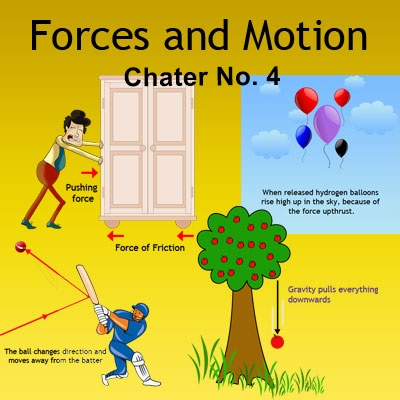# F.Sc. Physics First Year Chapter No. 3: Force and Motion Free Download

Chapter No. 3: Force and Motion Complete Notes
Here we are offering F.Sc. Physics first year notes Chapter No. 3 (Force and Motion). These 11th class Physics chapter no. 3 notes will provide a complete and accurate explanations as well as answers for each topic and question. Important long questions, solved exercises, important short questions and answers and board multiple choice questions are added in these notes. Students can access these notes given here for free and these are the premier study materials that will prove to be useful in preparing exams and scoring higher marks in the exams.
This chapter is divided into four parts:Theory
Solved Exercise
Solved Mathematical Problems
Multiple choice questions
These notes are very helpful to prepare Physics paper of F.Sc. Part 1 for Federal Board, Multan Board, Faisalabad Board, Sargodha Board, Gujranwala Board, DG Khan Board, Rawalpindi Board or any other board of Punjab, Pakistan.
Notes of Chapter 03: Motion and Force of “Physics XI” for F.Sc. Part 1 published by Punjab Textbook Board, Lahore. These notes are very helpful to prepare Physics paper of F.Sc. Part 1 for Federal Board and all Punjab boards of Pakistan.
Major Concepts
➧Motion
Displacement
Velocity
Average Velocity
Instantaneous Velocity
Acceleration
Average Acceleration
Instantaneous Acceleration
Uniform Acceleration
Review of Equations of Uniformly Accelerated Motion
Acceleration due to Gravity
Newton's Laws of Motion
Momentum
Impulse
Isolated System
Laws of Conservation of Momentum
Collision
Elastic Collision in one Dimension
Force due to Water Flow
Momentum and Explosive Forces
Rocket Propulsion
Projectile Motion
Applications to Ballistic Missiles
First year Physics notes of chapter no. 3 are given below. Students can download these notes in PDF format.
Click the following links to download Physics first year (F.Sc. Part-1) chapter no. 3 notes.
Exercise Short Questions
Solved mathematical problems
Board multiple choice questions
Complete chapter notes

You may also like these notes
You may also like these guess papers

For comprehensive notes of Physics for matric and intermediate classes, visit the following website